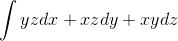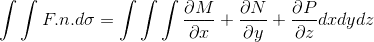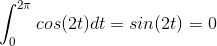# Integral on curve

solveon curve c that is the bigest circle of sphere [URL]http://latex.codecogs.com/gif.latex?(x-1)^2+(y-1)^2+(z-1)^2=1[/URL]

Last edited by a moderator:

What have you tried until now? Do you know how to do integrals on a curve in a higher-dimensional space (like, for example, integrals on a curve in the plane)?

i know F=(M,N,P)=(yz,xz,xy) and c is on closed curve i think i should usebut the euqals is zero i'm not sure . plaese help

This cannot be since dσ is two-dimensional, while dx dy dz is three-dimensional, and your integral is a line integral, i.e. one-dimensional. So no.

If you have an integral over a curve (a line integral), this is per definition
$$\int_C f_1(x, y, ...) \mathrm{d} x + f_2(x, y, ...) \mathrm{d} y + ... = \int_C f_1(x(t), y(t), ...) \frac{\partial x(t)}{\partial t} \mathrm{d} t + f_2(x(t), y(t), ...) \frac{\partial y(t)}{\partial t} \mathrm{d} t + ...$$, where x(t), y(t), ... describe your curve. Can you give me x(t), y(t) and z(t) for your curve?

if [URL]http://latex.codecogs.com/gif.latex?x^2+y^2+z^2=1[/URL] we get x(t)=cost , y(t)=sint and z(t)=1 but for [URL]http://latex.codecogs.com/gif.latex?(x-1)^2+(y-1)^2+(z-1)^2=1[/URL] i'm not know ??

Last edited by a moderator:
What if you try
$$x(t) = 1+cos(t) , y(t) = 1+sin(t) , z(t) = 1$$
Then you get
$$(1 + cos(t) -1)^{2} + (1 + sin(t) - 1)^{2} + (1-1)^{2} = 1$$
thus
$$cos^{2}(t) + sin^{2}(t) = 1$$

if [URL]http://latex.codecogs.com/gif.latex?x^2+y^2+z^2=1[/URL] we get x(t)=cost , y(t)=sint and z(t)=1 but for [URL]http://latex.codecogs.com/gif.latex?(x-1)^2+(y-1)^2+(z-1)^2=1[/URL] i'm not know ??

Sure you meant z = 0 there. Just as Clever-Name has said, adding 1 to each coordinate then gives you the curve for your problem. (If it is your curve, I'm not quite sure what a "bigest circle of sphere" is.).

Then just plug everything into the integral, you should get something like
$$\int_{0}^{2 \pi} \left[ - \sin t - \sin^2 t + \cos t + \cos^2 t \right] \mathrm{d} t = 0$$.

Last edited by a moderator:
HallsofIvy
Homework Helper
The "biggest circle on a sphere" is simply as "great circle" and there are an infinite number of them. The simplest thing to do is to take the great circle that is parallel to the xy-plane, given by x= 1+ cos(t), y= 1+ sin(t), z= 1.
Then dx= -sin(t)dt, dy= cos(t)dt, and dz= 0. yz= 1+ sin(t), xz= 1+ cos(t), and xy= 1+ sin(t)+ cos(t)+ sin(t)cos(t) but since that will be multiplied by dz= 0, it doesn't matter. The integral will be
$$\int_{t=0}^{2\pi} [-(1+ sin(t))sin(t)+ (1+ cos(t))cos(t)]dt$$
The identity $cos^2(t)- sin^2(t)= cos(2t)$ might be helpful.

I said there are an infinite number of great circles but it should be evident from the symmetry that they all give the same integral.

The "biggest circle on a sphere" is simply as "great circle" and there are an infinite number of them. The simplest thing to do is to take the great circle that is parallel to the xy-plane, given by x= 1+ cos(t), y= 1+ sin(t), z= 1.
Then dx= -sin(t)dt, dy= cos(t)dt, and dz= 0. yz= 1+ sin(t), xz= 1+ cos(t), and xy= 1+ sin(t)+ cos(t)+ sin(t)cos(t) but since that will be multiplied by dz= 0, it doesn't matter. The integral will be
$$\int_{t=0}^{2\pi} [-(1+ sin(t))sin(t)+ (1+ cos(t))cos(t)]dt$$
The identity $cos^2(t)- sin^2(t)= cos(2t)$ might be helpful.

I said there are an infinite number of great circles but it should be evident from the symmetry that they all give the same integral.